# Make a decision tree from 0 with Python and understand it (4. Data structure)

** Create and understand decision trees from scratch in Python ** 1. Overview-2. Python Program Basics-3. Data Analysis Library Pandas --4 Data Structure

For learning AI (machine learning) and data mining, we will understand by creating a decision tree from scratch in Python.

## 4.1 Data structure

A data structure is a representation of how individual data are arranged.

### Array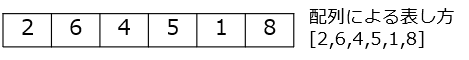The individual data are lined up in a row. To identify one piece of data, you need one identifier, for example, what number of data.

``````#Implementation example of array in python
a = [2,6,4,5,1,8]
``````

### Table, two-dimensional array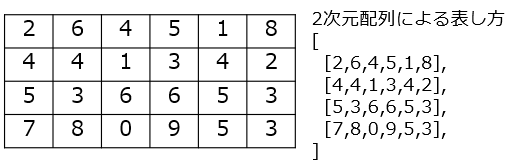There are multiple columns of data, with individual data lined up on a plane. To identify one piece of data, you need two identifiers, like the number in the column.
``````#Implementation example of table in python
a = [
[2,6,4,5,1,8],
[4,4,1,3,4,2],
[5,3,6,6,5,3],
[7,8,0,9,5,3],
]
``````

### Tree, tree structure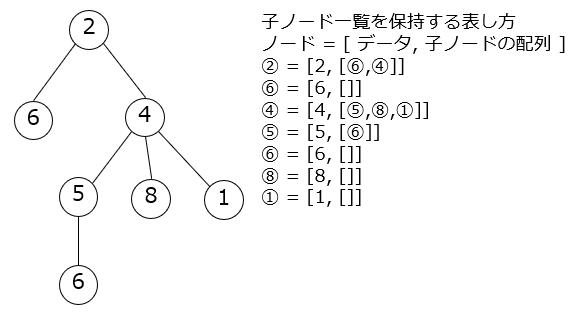It is a data structure that connects individual data with a line. However, the line does not circulate, for example, when considering the route from one data to another, the one with one route is the data of the tree structure. It is often illustrated as a tree that extends from top to bottom, as shown below. Lines are called edges and branches, data is called nodes, data with lines below them is called trunks and nodes, data without lines is called leaves and leaves, and top data is called root nodes and roots. I will call you. The line may be one-way with an arrow.

``````#Implementation example of tree in python Holds a list of child nodes.
# [value,Array of child node list]For example, the upper tree is implemented from top to bottom and from left to right as follows.
#Other than this implementation method, there are methods such as using a class and holding a parent node.
tree = \
[2,[
[6,[]],
[4,[
[5,[
[6,[]],
]],
[8,[]],
[1,[]],
]],
]]

#Tree structure display function
def tstr(node,indent=""):
print(indent+str(node))
for c in node: #Loop on child node
tstr(c,indent+"+-")
tstr(tree)
#output
# 2
# +-6
# +-4
# +-+-5
# +-+-+-6
# +-+-8
# +-+-1

#If you don't want to make the whole tree at once, but one by one
#Create all leaf nodes that have no child nodes. The variable name is the number from the row (column) and the left.
n10 = [6,[]]
n21 = [8,[]]
n22 = [1,[]]
n40 = [6,[]]
#Create all the child nodes in order from the generated node.
n20 = [5,[n40]]
n11 = [4,[n20,n21,n22]]
n00 = [2,[n10,n11]]

#Display tree structure, display the specified node as the root node.
tstr(n11)
#output
# 4
# +-5
# +-+-6
# +-8
# +-1
``````

### Network, graph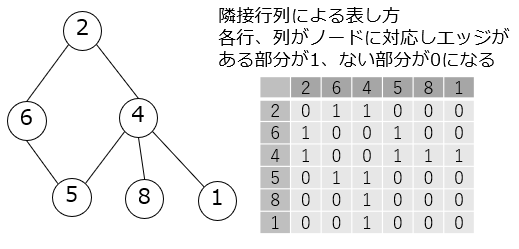It is a data structure that connects data with a circular line with a line. Similar to the tree structure, the line can also be one-way with an arrow. Individual data are called nodes, and lines are called edges. Since there is often no starting point for data like a tree structure, we don't often call routes, trunks, leaves, etc.
``````#Implementation example of network in python
import pandas as pd

#It is assumed that the node name and the value match.
#If the name and value do not match, you need data to subtract the value from the name.
nodes = [2,6,4,5,8,1]
#Define the connection status of nodes in the form of a matrix. Node 2(The first line)From node 6(2nd line)If there is an edge
#The 1-by-2 value of the matrix is 1, and 0 if there are no edges. This matrix is called an adjacency matrix.
df = pd.DataFrame(
[
# 2,6,4,5,8,Is there a connection to one node?
[ 0,1,1,0,0,0], #From 2 nodes
[ 1,0,0,1,0,0], #From 6 nodes
[ 1,0,0,1,1,1], #From 4 nodes
[ 0,1,1,0,0,0], #From 5 nodes
[ 0,0,1,0,0,0], #From 8 nodes
[ 0,0,1,0,0,0], #From one node
],columns=nodes,index=nodes)
print(df)
#output
#    2  6  4  5  8  1
# 2  0  1  1  0  0  0
# 6  1  0  0  1  0  0
# 4  1  0  0  1  1  1
# 5  0  1  1  0  0  0
# 8  0  0  1  0  0  0
# 1  0  0  1  0  0  0

#The network is drawn by matplotlib and a library called networkx.
import matplotlib.pyplot as plt
import networkx as nx
plt.figure(figsize=(4,4))
plt.axis("off")
plt.show()
``````

Network output example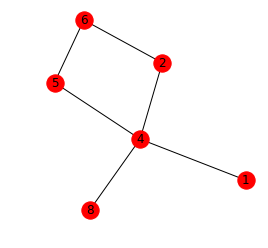## 4.2 Example of implementation of decision tree by python

Decision trees, as the name implies, can be represented by a tree structure. The data held by the node includes the rules for branching and the data list that reaches this node in the decision tree, in addition to the list of child nodes that have a tree structure.

Place an empty node on the root node and associate all the data as shown below. The [...] number attached to the node represents the data number of the original data from which this decision tree is created. Then, from the root node, only the data that meets the conditions of the child node can be expressed as going down the tree. The nodes in the decision tree that go golf and don't go golf can be found by looking at the data associated with the node.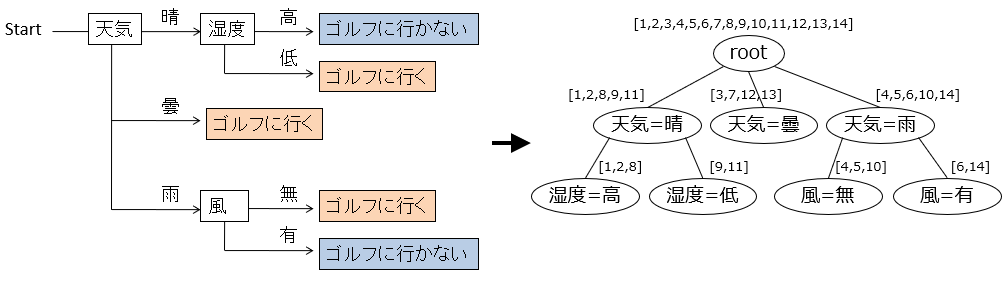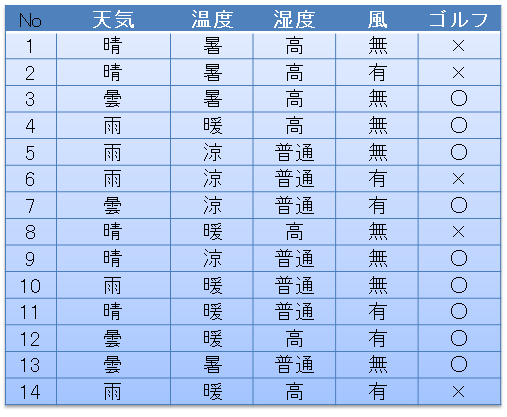The implementation in python is as follows, for example. One node is an associative array, name is a character representation of the condition of that node, df is the data associated with that node, and edges is a list of child nodes.

``````#Tree structure data
tree = {
# name:This node(stem)s name
"name":"decision tree "+df0.columns[-1]+" "+str(cstr(df0.iloc[:,-1])),
# df:Data associated with this node
"df":df0,
# edges:Edge coming out of this node(branch)In the list, if the leaf node has no edge below, it will be an empty array.
"edges":[],
}
``````

The function tstr that characterizes this tree structure looks like this:

``````#Lambda expression for value distribution, argument is pandas.Series, return value is an array containing the number of each value
#Input s to value_counts()Get the frequency of each value with, and loop items of dictionary type data()To call.
#sorted sorts in ascending order of frequency so that the output result does not change with each execution.
#And the element is the key(k)And value(v)Generate an array that is a character string of.
cstr = lambda s:[k+":"+str(v) for k,v in sorted(s.value_counts().items())]

#Method to convert tree to characters, argument is tree:Tree data structure, indent:Indentation on child nodes,
#The return value is a character representation of tree. This method is called recursively to convert everything in the tree to characters.
def tstr(tree,indent=""):
#Create a character representation for this node. If this node is a leaf node(The number of elements in the edges array is 0)To
#Characterize the frequency distribution in the last column of the data df associated with the tree.
s = indent+tree["name"]+str(cstr(tree["df"].iloc[:,-1]) if len(tree["edges"])==0 else "")+"\n"
#Loop on all edges from this node.
for e in tree["edges"]:
#Add the character representation of the child node to the character representation of this node.
#For indent, add more characters to the indent of this node.
s += tstr(e,indent+"  ")
pass
return s
``````

The decision tree transcribed by this tstr function looks like this: The root node shows the character (decision tree golf) set when the tree variable was first created and the frequency distribution of going / not going to golf for all data. In each node below it, the rules used for branching and, in the case of leaf nodes, the frequency distribution of going / not going golf in the data associated with that node (eg ['○: 2']). Is displayed.

``````decision tree golf['×:5', '○:9']
weather=Fine
Humidity=usually['○:2']
Humidity=High['×:3']
weather=Cloudy['○:4']
weather=rain
Wind=Yes['×:2']
Wind=Nothing['○:3']
``````